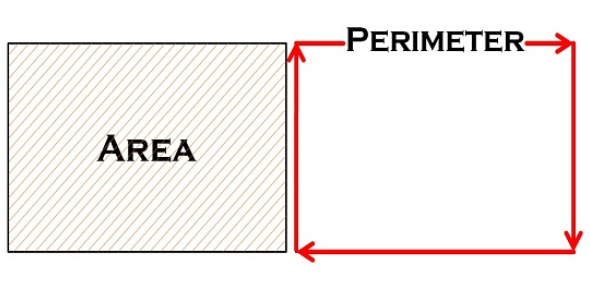Can You Pass This Toughest Test Of Area And Perimeter?

10 Questions | Total Attempts: 5905SettingsThis quiz has been designed to examine the mathematical concepts of length, Area and perimeter. Complete the quiz to show your mastery level for finding the area and perimeter of a given object. So, let's try out the quiz. All the best!

• 1.
A basketball court has a length of 4 feet and a height of 6 feet. What is the area of this basketball court?
• A.

Ten feet

• B.

Ten square feet

• C.

Twenty four square feet

• D.

Twenty four feet

• 2.
What is the perimeter of the basketball court in question 1? (length is 4 feet and height is 6 feet)
• A.

20 feet

• B.

24 square feet

• C.

20 square feet

• D.

24 feet

• 3.
In Frank's backyard is 20 square feet and he wants the basketball court from question 1 to be placed in his backyard, does Frank have enough room? (hint: you need to know the area)
• A.

Yes, The backyard is big enough because the backyard and the basketball court both equal 20 square feet.

• B.

No, the backyard is not big enough because the basketball court is 24 square feet.

• 4.
If Mrs. Santora's room is 5 feet by 5 feet, what is the area of her room?
• A.

25 square feet

• B.

10 square feet

• C.

10 feet

• D.

25 feet

• 5.
1. Choose the correct formula for finding the perimeter of any shape.
• A.

Length times width

• B.

Base times height

• C.

Base times height divided by two

• D.

• 6.
What is the formula for finding the volume of a rectangular prism?
• A.

Base times height

• B.

• C.

Length plus width plus height

• D.

Length times width times height

• 7.
Do you add, subtract, multiply or divide the sides to find perimeter?
• A.

• B.

Subtract

• C.

Multiply

• D.

Divide

• 8.
To calculate the amount of carpeting needed for your bedroom, which of the following should you find:
• A.

Area

• B.

Perimeter

• C.

Surface Area

• D.

Volume

• 9.
Find the perimeter of the figure?
• A.

16 cm

• B.

64 sq cm

• C.

64 cm

• D.

32 cm

• 10.
Find the perimeter of the figure.
• A.

42 cm

• B.

124 cm

• C.

64 cm

• D.

45 cm

Related TopicsBack to top